## 5.258. min_size_full_zero_stretch

Origin

Derived from the unit commitment problem

Constraint

$\mathrm{𝚖𝚒𝚗}_\mathrm{𝚜𝚒𝚣𝚎}_\mathrm{𝚏𝚞𝚕𝚕}_\mathrm{𝚣𝚎𝚛𝚘}_\mathrm{𝚜𝚝𝚛𝚎𝚝𝚌𝚑}\left(\mathrm{𝙼𝙸𝙽𝚂𝙸𝚉𝙴},\mathrm{𝚅𝙰𝚁𝙸𝙰𝙱𝙻𝙴𝚂}\right)$

Arguments
 $\mathrm{𝙼𝙸𝙽𝚂𝙸𝚉𝙴}$ $\mathrm{𝚒𝚗𝚝}$ $\mathrm{𝚅𝙰𝚁𝙸𝙰𝙱𝙻𝙴𝚂}$ $\mathrm{𝚌𝚘𝚕𝚕𝚎𝚌𝚝𝚒𝚘𝚗}\left(\mathrm{𝚟𝚊𝚛}-\mathrm{𝚍𝚟𝚊𝚛}\right)$
Restrictions
 $\mathrm{𝙼𝙸𝙽𝚂𝙸𝚉𝙴}\ge 0$ $\mathrm{𝙼𝙸𝙽𝚂𝙸𝚉𝙴}\le |\mathrm{𝚅𝙰𝚁𝙸𝙰𝙱𝙻𝙴𝚂}|$ $\mathrm{𝚛𝚎𝚚𝚞𝚒𝚛𝚎𝚍}$$\left(\mathrm{𝚅𝙰𝚁𝙸𝙰𝙱𝙻𝙴𝚂},\mathrm{𝚟𝚊𝚛}\right)$
Purpose

Given an integer $\mathrm{𝙼𝙸𝙽𝚂𝙸𝚉𝙴}$ and a sequence of variables $\mathrm{𝚅𝙰𝚁𝙸𝙰𝙱𝙻𝙴𝚂}$ enforce $\mathrm{𝙼𝙸𝙽𝚂𝙸𝚉𝙴}$ to be greater than or equal to the size of the smallest full stretch of zero of $\mathrm{𝚅𝙰𝚁𝙸𝙰𝙱𝙻𝙴𝚂}$ or to $|\mathrm{𝚅𝙰𝚁𝙸𝙰𝙱𝙻𝙴𝚂}|$ if no full stretch of zero exists.

A stretch of zero is a maximum sequence of zero, while a full stretch of zero is a stretch of zero that is neither located at the leftmost nor at the rightmost border of the sequence of variables $\mathrm{𝚅𝙰𝚁𝙸𝙰𝙱𝙻𝙴𝚂}$. The size of a stretch of zero is the number of zero of the stretch.

Example
$\left(2,〈0,2,0,0,0,2,1,0,0,3〉\right)$

Figure 5.258.1 shows the smallest full stretch of zero associated with the example. The $\mathrm{𝚖𝚒𝚗}_\mathrm{𝚜𝚒𝚣𝚎}_\mathrm{𝚏𝚞𝚕𝚕}_\mathrm{𝚣𝚎𝚛𝚘}_\mathrm{𝚜𝚝𝚛𝚎𝚝𝚌𝚑}$ constraint holds since the size of the smallest full stretch of zero of the sequence $0200021003$ is greater than or equal to 2.

##### Figure 5.258.1. Illustration of the Example slot: smallest full stretch of zero in bold and red ($\mathrm{𝙼𝙸𝙽𝚂𝙸𝚉𝙴}=2$); note that the leftmost stretch of zero of size 1 is ignored since it is located at one of the two extremities of the sequence $0200021003$.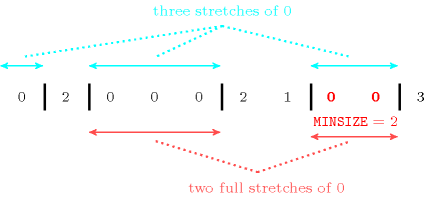Typical
 $|\mathrm{𝚅𝙰𝚁𝙸𝙰𝙱𝙻𝙴𝚂}|>2$ $\mathrm{𝚛𝚊𝚗𝚐𝚎}$$\left(\mathrm{𝚅𝙰𝚁𝙸𝙰𝙱𝙻𝙴𝚂}.\mathrm{𝚟𝚊𝚛}\right)>1$ $|\mathrm{𝚅𝙰𝚁𝙸𝙰𝙱𝙻𝙴𝚂}|-$$\mathrm{𝚊𝚖𝚘𝚗𝚐}_\mathrm{𝚍𝚒𝚏𝚏}_\mathtt{0}$$\left(\mathrm{𝚅𝙰𝚁𝙸𝙰𝙱𝙻𝙴𝚂}.\mathrm{𝚟𝚊𝚛}\right)>1$
Symmetries
• Items of $\mathrm{𝚅𝙰𝚁𝙸𝙰𝙱𝙻𝙴𝚂}$ can be reversed.

• An occurrence of a value of $\mathrm{𝚅𝙰𝚁𝙸𝙰𝙱𝙻𝙴𝚂}.\mathrm{𝚟𝚊𝚛}$ that is different from 0 can be replaced by any other value that is also different from 0.

Counting
 Length ($n$) 2 3 4 5 6 7 8 Solutions 9 82 1137 19026 364033 7850291 188987201

Number of solutions for $\mathrm{𝚖𝚒𝚗}_\mathrm{𝚜𝚒𝚣𝚎}_\mathrm{𝚏𝚞𝚕𝚕}_\mathrm{𝚣𝚎𝚛𝚘}_\mathrm{𝚜𝚝𝚛𝚎𝚝𝚌𝚑}$: domains $0..n$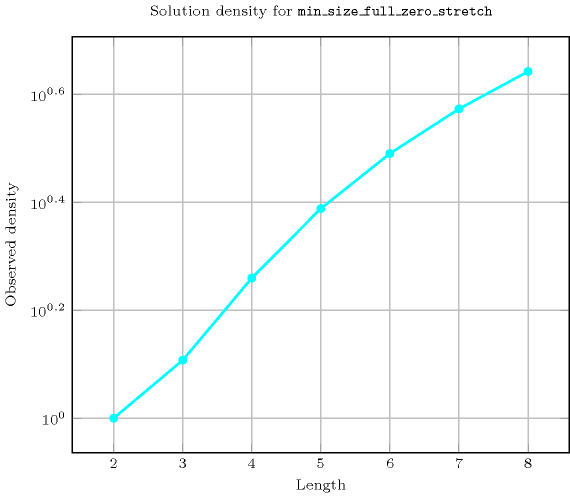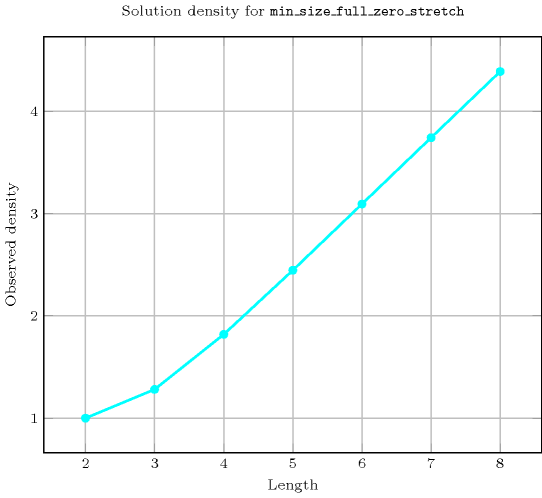Length ($n$)2345678
Total9821137190263640337850291188987201
 Parameter value

1-916025754507288244119330432
29917628754993296667220958912
3-6417629005043697539421117888
4--62529005047297617821132416
5---77765047297622721133568
6----11764997622721133632
7-----209715221133632
8------43046721

Solution count for $\mathrm{𝚖𝚒𝚗}_\mathrm{𝚜𝚒𝚣𝚎}_\mathrm{𝚏𝚞𝚕𝚕}_\mathrm{𝚣𝚎𝚛𝚘}_\mathrm{𝚜𝚝𝚛𝚎𝚝𝚌𝚑}$: domains $0..n$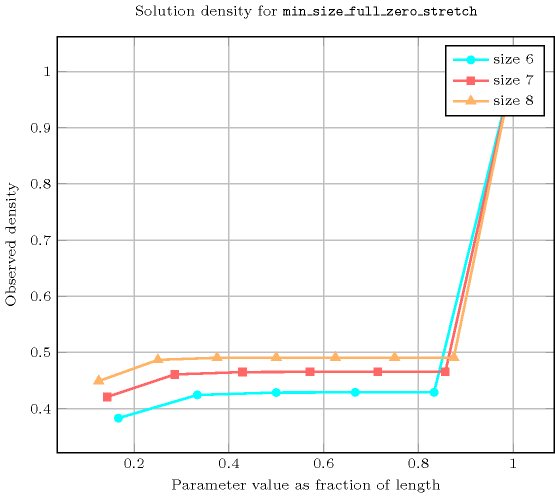Figure 5.258.2 depicts the automaton associated with the $\mathrm{𝚖𝚒𝚗}_\mathrm{𝚜𝚒𝚣𝚎}_\mathrm{𝚏𝚞𝚕𝚕}_\mathrm{𝚣𝚎𝚛𝚘}_\mathrm{𝚜𝚝𝚛𝚎𝚝𝚌𝚑}$ constraint.
##### Figure 5.258.2. Automaton of the $\mathrm{𝚖𝚒𝚗}_\mathrm{𝚜𝚒𝚣𝚎}_\mathrm{𝚏𝚞𝚕𝚕}_\mathrm{𝚣𝚎𝚛𝚘}_\mathrm{𝚜𝚝𝚛𝚎𝚝𝚌𝚑}$ constraint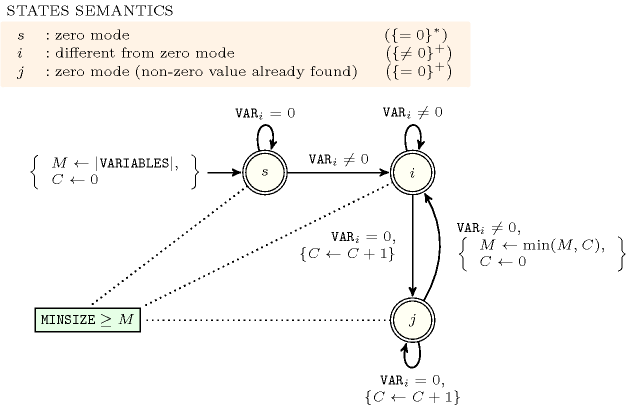##### Figure 5.258.3. Hypergraph of the reformulation corresponding to the automaton (with two counters) of the $\mathrm{𝚖𝚒𝚗}_\mathrm{𝚜𝚒𝚣𝚎}_\mathrm{𝚏𝚞𝚕𝚕}_\mathrm{𝚣𝚎𝚛𝚘}_\mathrm{𝚜𝚝𝚛𝚎𝚝𝚌𝚑}$ constraint where $l=|\mathrm{𝚅𝙰𝚁𝙸𝙰𝙱𝙻𝙴𝚂}|$ (since all states of the automaton are accepting there is no restriction on the last variable ${Q}_{n}$)